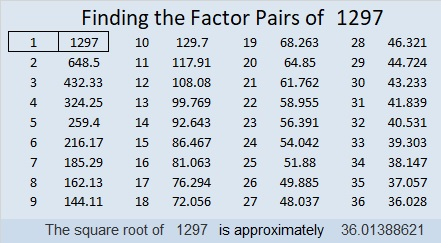# 1297 Mystery

Today’s puzzle has 12 clues, but it still presents quite a mystery. Will you be able to figure out where the factors from 1 to 12 go in the 1st column and the top row of the puzzle, or will you let this mystery stump you?Print the puzzles or type the solution in this excel file: 12 factors 1289-1299

Now I’ll share a little bit of information about the number 1297:

• 1297 is a prime number.
• Prime factorization: 1297 is prime.
• The exponent of prime number 1297 is 1. Adding 1 to that exponent we get (1 + 1) = 2. Therefore 1297 has exactly 2 factors.
• Factors of 1297: 1, 1297
• Factor pairs: 1297 = 1 × 1297
• 1297 has no square factors that allow its square root to be simplified. √1297 ≈ 36.01389

How do we know that 1297 is a prime number? If 1297 were not a prime number, then it would be divisible by at least one prime number less than or equal to √1297 ≈ 36.01. Since 1297 cannot be divided evenly by 2, 3, 5, 7, 11, 13, 17, 19, 23, 29 or 31, we know that 1297 is a prime number.1297 is the sum of two squares:
36² +  1² = 1297

1297 is the hypotenuse of a Pythagorean triple:
72-1295-1297 calculated from 2(36)(1), 36² –  1², 36² +  1²

Here’s another way we know that 1297 is a prime number: Since its last two digits divided by 4 leave a remainder of 1, and 36² + 1² = 1297 with 36 and 1 having no common prime factors, 1297 will be prime unless it is divisible by a prime number Pythagorean triple hypotenuse less than or equal to √1297 ≈ 36.01. Since 1297 is not divisible by 5, 13, 17, or 29, we know that 1297 is a prime number.